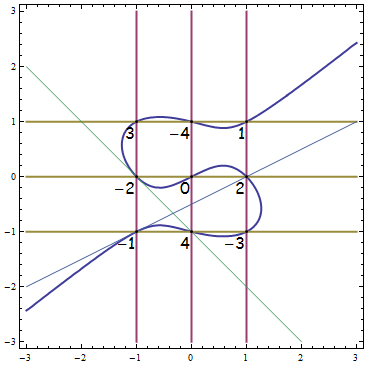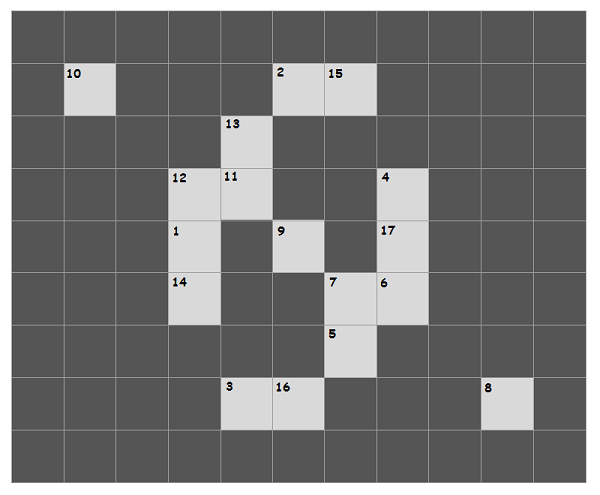# Noughts and Crosses

Firstly, the results for the Balkan Mathematical Olympiad have been published. Consequently, the preliminary UK IMO squad is now larger than ever before, comprising ten people (six team members and four reserves, in some indeterminate order).

You are probably aware of the game of Noughts and Crosses (alternatively referred to as tic-tac-toe), in which one attempts to seize three collinear squares whilst attempting to prevent one’s opponent from doing likewise. What is less well-known, albeit mentioned in the Royal Institution Christmas Lectures several years ago, is that it’s entirely equivalent to the following problem:

We begin with the numbers {1, 2, 3, … 9}. Players alternate turns, taking an available (unclaimed) number. The first player to obtain three numbers which sum to 15 is declared the winner.

To demonstrate their equivalence, it suffices to show that we can label the squares from 1 to 9, inclusive, such that every sum to 15 corresponds to a unique line, and vice-versa. This is accomplished by the following magic square:It is immediate from the definition of the magic square that all lines (sets of three collinear squares) consist of three distinct numbers in the range {1, 2, …, 9} summing to 15. What is less obvious is the converse, that every such set is represented by a line. I only just realised the real reason behind this, which hitherto seemed to be a mere coincidence. Firstly, we decrement each of the numbers by 5, so that all line sums are zero.Now, think of these as points rather than squares. For convenience, we shall allow 0 to be the origin. On the diagram below are the curves x(x − 1)(x + 1) = 0, y(y − 1)(y + 1) = 0, and the linear combination x(x − 1)(x + 1) = 2y(y − 1)(y + 1). The latter must also pass through all nine grid points, being a combination of the other two.This is an elliptic curve, so it admits a well-known Abelian group structure. We chose the coefficient 2 in the equation for the curve specifically so that the tangent at -1 intersects the elliptic curve again at 2; similarly, the tangent at -2 intersects the curve again at 4. From this and the remaining lines, it is easy to see that the elliptic curve group operation corresponds to ordinary integer addition. And we know that three points on an elliptic curve sum to zero if and only if they are collinear (c.f. my elliptic curve calculator, which was recently made into a video by James Grime of DAMTP):